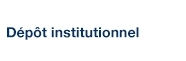# On the universality of crossing probabilities in two-dimensional percolation

Langlands, R. P.; Pichet, Claude; Pouliot, Ph. et Saint-Aubin, Y. (1992). « On the universality of crossing probabilities in two-dimensional percolation ». Journal of Statistical Physics, 67(3-4), pp. 553-574.

Fichier(s) associé(s) à ce document :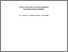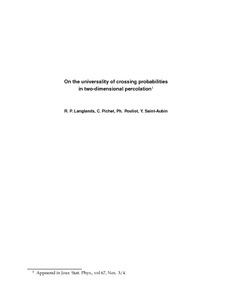Prévisualisation
PDF
Télécharger (212kB)

## Résumé

Six percolation models in two dimensions are studied: percolation by sites and by bonds on square, hexagonal, and triangular lattices. Rectangles of widtha and heightb are superimposed on the lattices and four functions, representing the probabilities of certain crossings from one interval to another on the sides, are measured numerically as functions of the ratioa/b. In the limits set by the sample size and by the conventions and on the range of the ratioa/b measured, the four functions coincide for the six models. We conclude that the values of the four functions can be used as coordinates of the renormalization-group fixed point.

Type: Article de revue scientifique Percolation in two dimensions, universality classes in percolation theory, renormalization group Faculté des sciences > Département de mathématiques Claude Pichet 25 avr. 2016 12:49 27 avr. 2016 19:30 http://archipel.uqam.ca/id/eprint/8280

## Statistiques

Voir les statistiques sur cinq ans...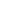RECHERCHER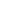PARCOURIR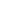LIBRE ACCÈS